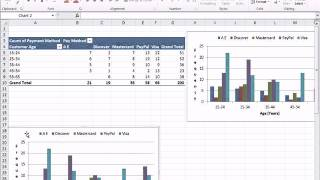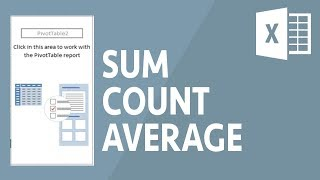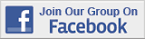Excel Tips & Tutorials:
Making Comparisons
 Suggest a Link Alphabetize Page Printer-Friendly List
It Has to Start Sometime
Watch and Learn
Watch and Learn
Watch and Learn
Watch and Learn
 Cross Tabulation With Frequencies and PercentagesShows how to calculate values and format PivotTable (15:12).Using SUM, COUNT and AVERAGE in PivotTablesSkills needed to make create mean comparison tables (9:51).Calculating Means and Standard Deviations in PivotTableUseful for creating mean comparison tables (7:34).Excel PivotTables Made EasyDemonstrates PivotTables with sales data (DV) and addresses common problems (13:17).Cross Tabulation With Frequencies and PercentagesShows how to calculate values and format PivotTable (15:12).Using SUM, COUNT and AVERAGE in PivotTablesSkills needed to make create mean comparison tables (9:51).Calculating Means and Standard Deviations in PivotTableUseful for creating mean comparison tables (7:34).Cross Tabulation With Frequencies and PercentagesShows how to calculate values and format PivotTable (15:12).Using SUM, COUNT and AVERAGE in PivotTablesSkills needed to make create mean comparison tables (9:51).Cross Tabulation With Frequencies and PercentagesShows how to calculate values and format PivotTable (15:12).Over 18,000 Members!